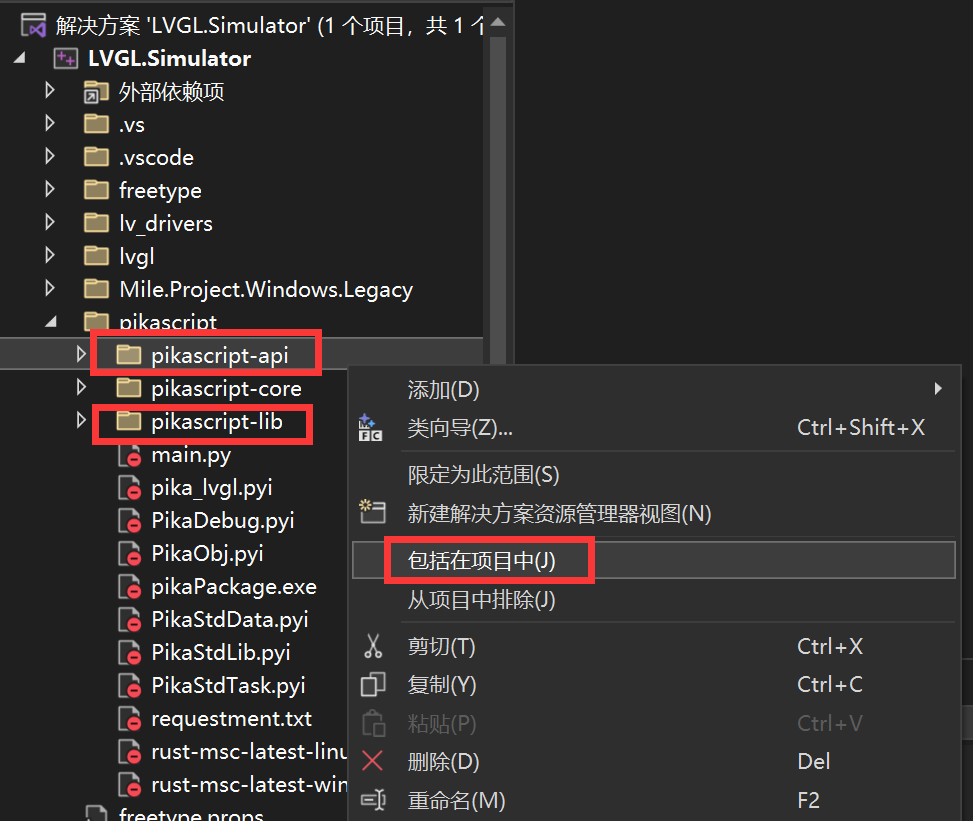# 2.6. 从 LVGL GUI 仿真工程开始

LVGL GUI 仿真工程提供了 PikaPython 和 LVGL 进行联合仿真的实验环境。

## 2.6.1. 获取工程

http://pikascript.com/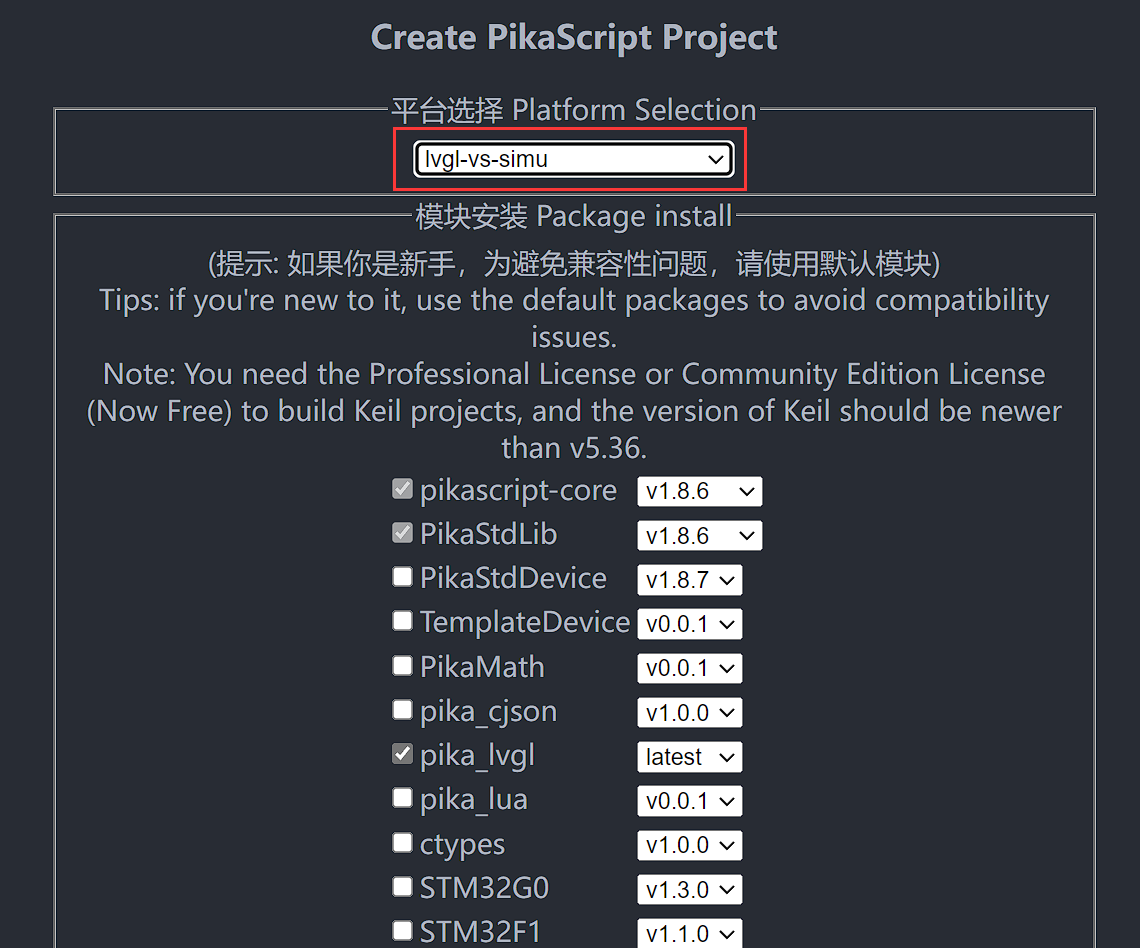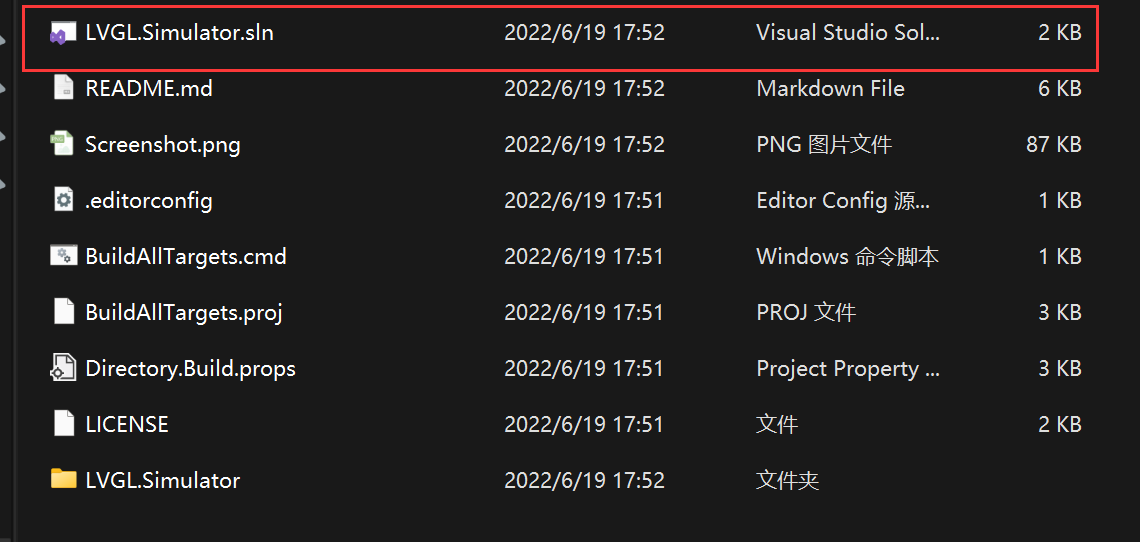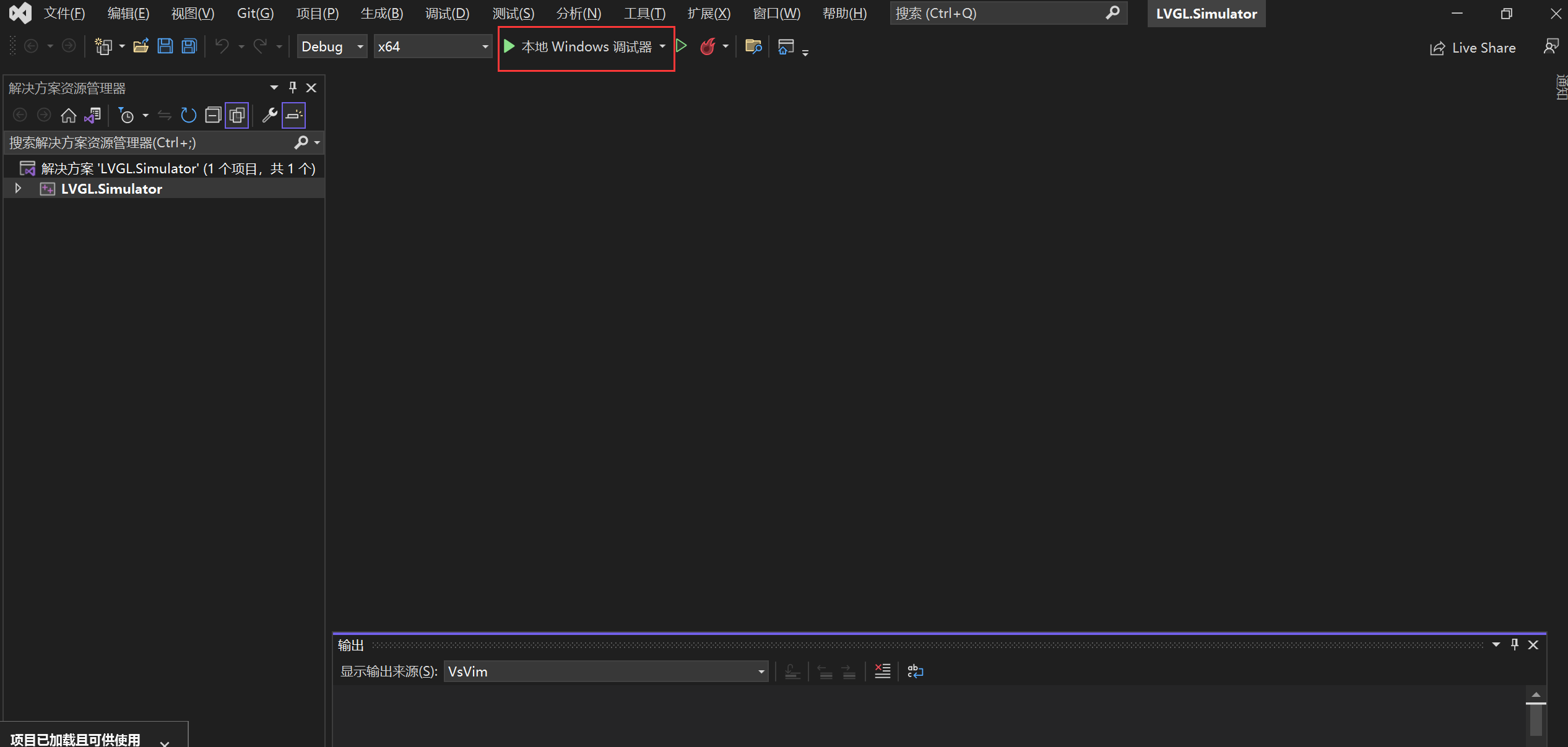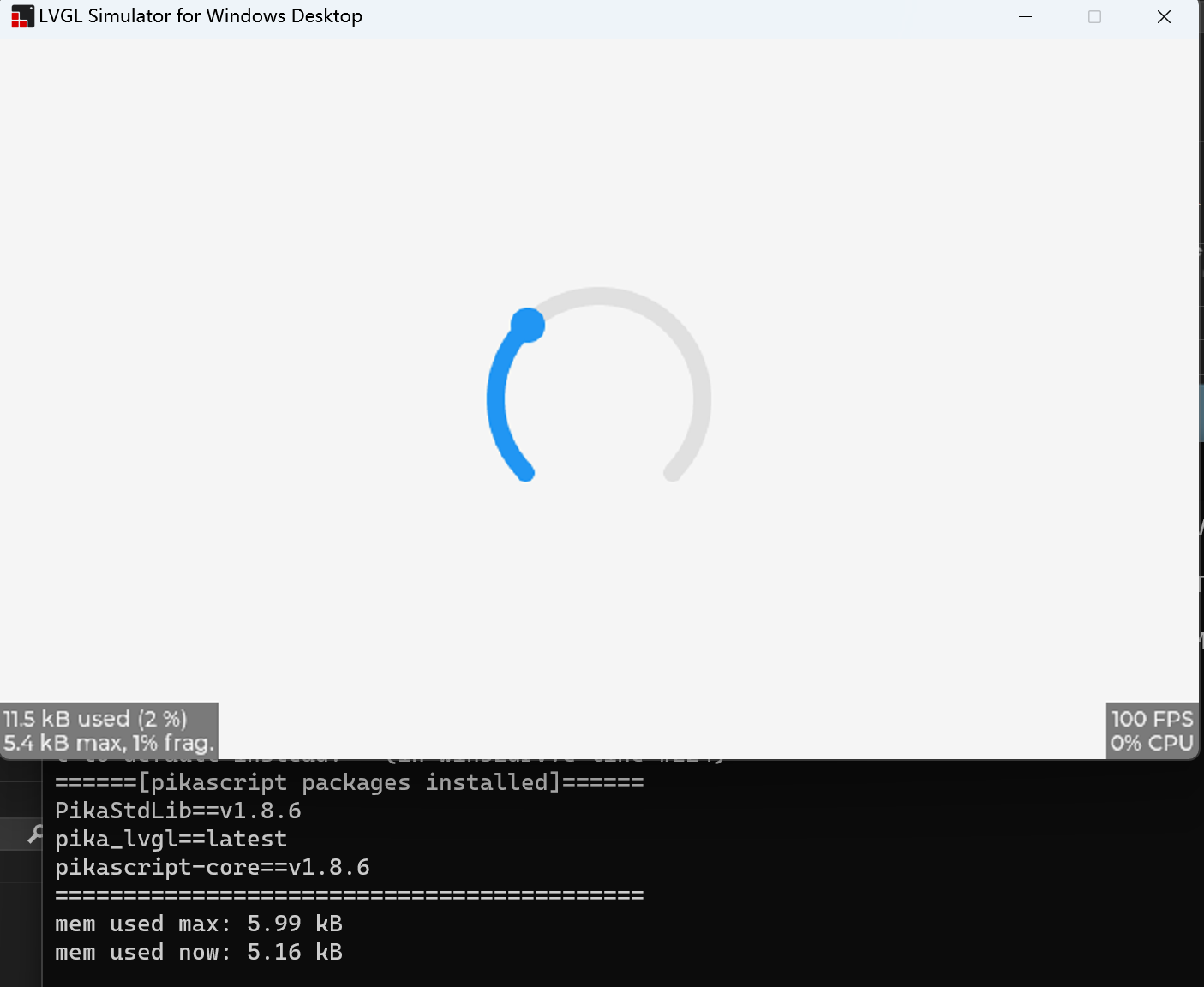## 2.6.2. 使用 Python 编程main.py 中的代码如下所示，工程在启动后就会运行这个 main.py

```# main.py
import pika_lvgl as lv
import PikaStdLib
mem = PikaStdLib.MemChecker()

# Create an Arc
arc = lv.arc(lv.scr_act())
arc.set_end_angle(200)
arc.set_size(150, 150)
arc.center()

print('mem used max: %0.2f kB' % (mem.getMax()))
print('mem used now: %0.2f kB' % (mem.getNow()))
```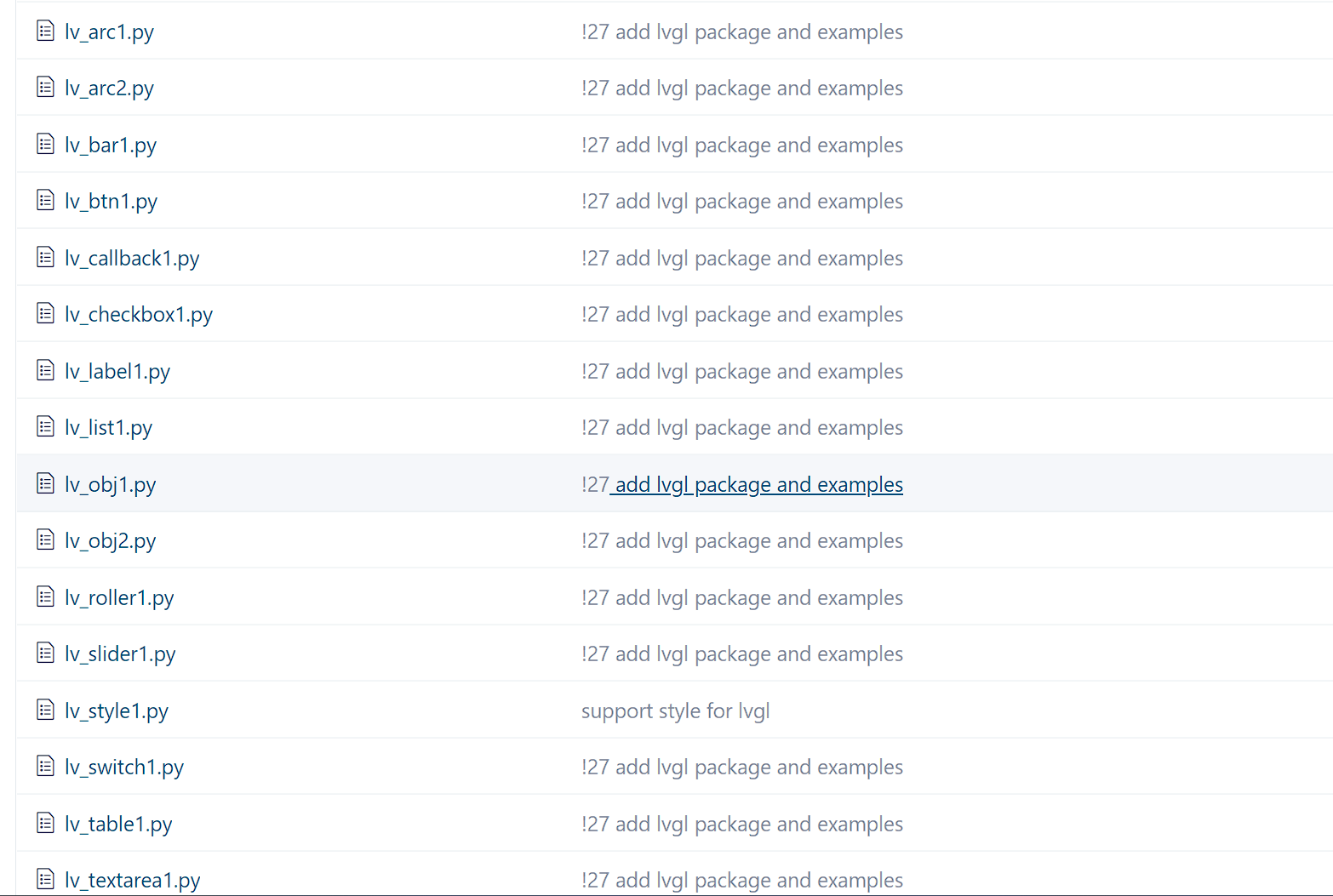```# lv_callback1.py
import pika_lvgl as lv
import PikaStdLib
mem = PikaStdLib.MemChecker()

def event_cb_1(evt):
print('in evt1')
print('mem used now: %0.2f kB' % (mem.getNow()))

def event_cb_2(evt):
print('in evt2')
print('mem used now: %0.2f kB' % (mem.getNow()))

btn1 = lv.btn(lv.scr_act())
btn1.align(lv.ALIGN.TOP_MID, 0, 10)
btn2 = lv.btn(lv.scr_act())
btn2.align(lv.ALIGN.TOP_MID, 0, 50)

print('mem used max: %0.2f kB' % (mem.getMax()))
print('mem used now: %0.2f kB' % (mem.getNow()))
```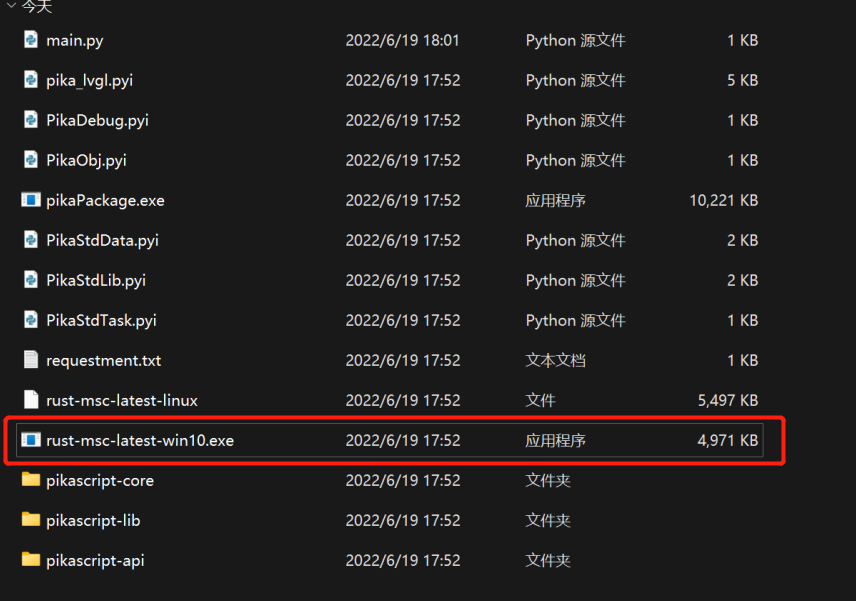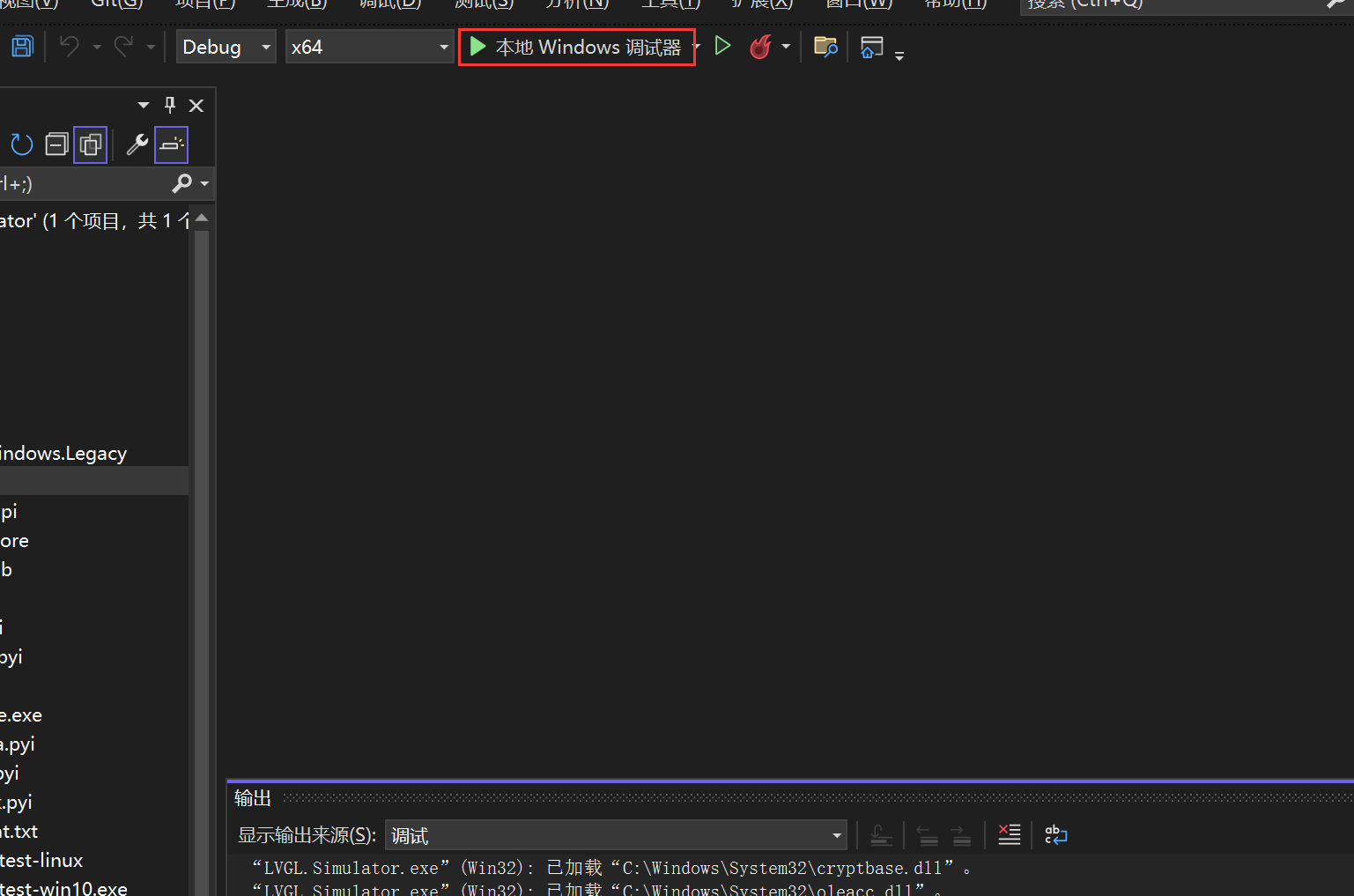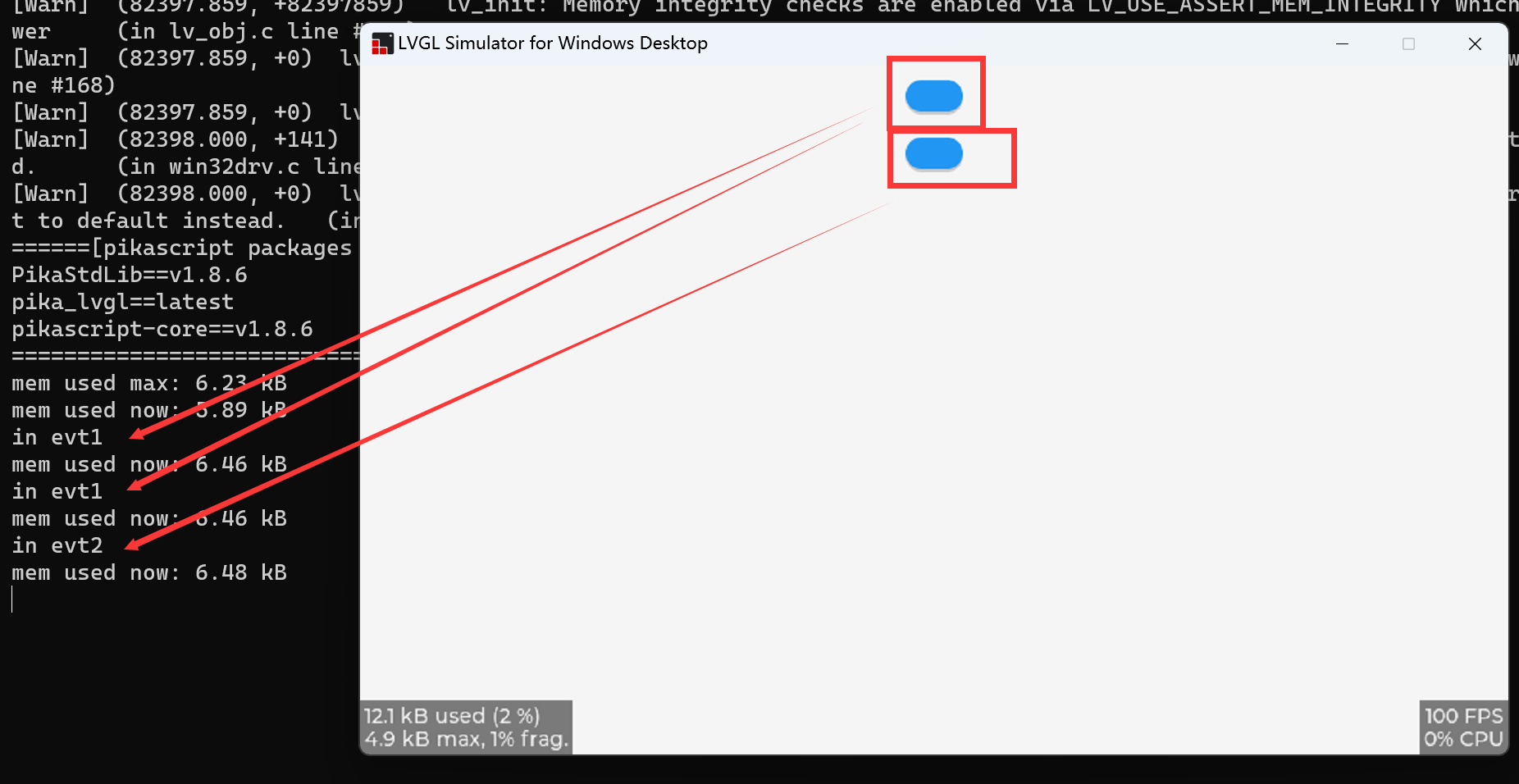## 2.6.3. 常见问题